Question

# 1) If the beta of the market index is 1.0 and the standard deviation of the...

1) If the beta of the market index is 1.0 and the standard deviation of the market index increases from 12% to 18%, what is the beta of the market index following this increase?

A. 0.8

B. 1.0

C. 1.2

D. 1.5

2) The security market line represents __________

A. the risk-return for all portfolios which can be constructed from the risk-free investment and the optimal risky portfolio

B. the relationship between beta and expected return

C. the relationship between an investment’s return and the return on an index

D. the relationship between an investment's return and the return on a negative beta portfolio

1) Beta of Market remains same irrespective of the standard deviation of the market.

Hence Option b Beta = 1.0

2) Option b relation between beta and expected return, Expected Return is on y axis and beta is on x axis is SML line

#### Earn Coins

Coins can be redeemed for fabulous gifts.

Similar Homework Help Questions
• ### Expected Portfolio Return Beta 22 0.8 A Market 173 1.0 D) Expected Portfolio Return Beta 30.28 1.8 A Market 198 1.0 If...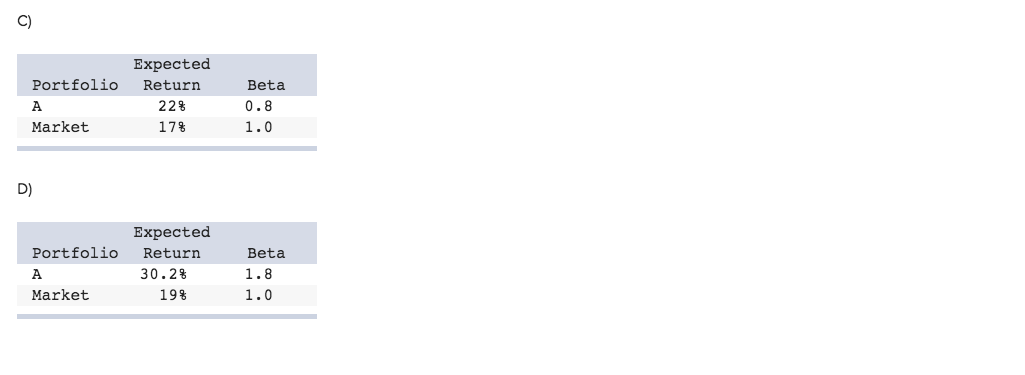Expected Portfolio Return Beta 22 0.8 A Market 173 1.0 D) Expected Portfolio Return Beta 30.28 1.8 A Market 198 1.0 If the simple CAPM is valid and all portfolios are priced correctly, which of the situations below is possible? Consider each situation independently, and assume the risk-free rate is 5% A) Expected Portfolio Return Beta 198 0.8 Market 198 1.0 B) Expected Standard Return Deviation Portfolio 228 88 A Market 17B 168

• ### Asset W has an expected return of 12.3 percent and a beta of 1.2. If the...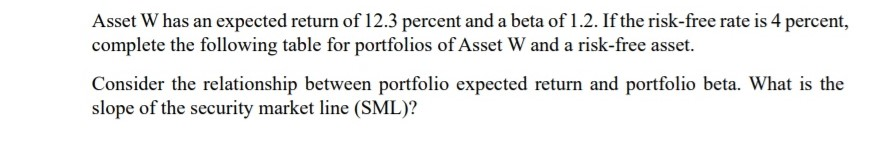Asset W has an expected return of 12.3 percent and a beta of 1.2. If the risk-free rate is 4 percent, complete the following table for portfolios of Asset W and a risk-free asset. Consider the relationship between portfolio expected return and portfolio beta. What is the slope of the security market line (SML)?

• ### 3. Suppose that the S&P 500, with a beta of 1.0, has an expected return of...

3. Suppose that the S&P 500, with a beta of 1.0, has an expected return of 13% and T-bills provide a risk-free return of 4%. a). What would be the expected return and beta of portfolios constructed from these two assets with weights in the S&P 500 of (i) 0; (ii) .25; (iii) .5; (iv) .75; (v) 1.0? b). On the basis of your answer to (a), what is the trade-off between risk and return, that is, how does expected...

• ### Compute the expected return, standard deviation, beta, and nonsystematic standard deviation of the portfolio. 4. Assume...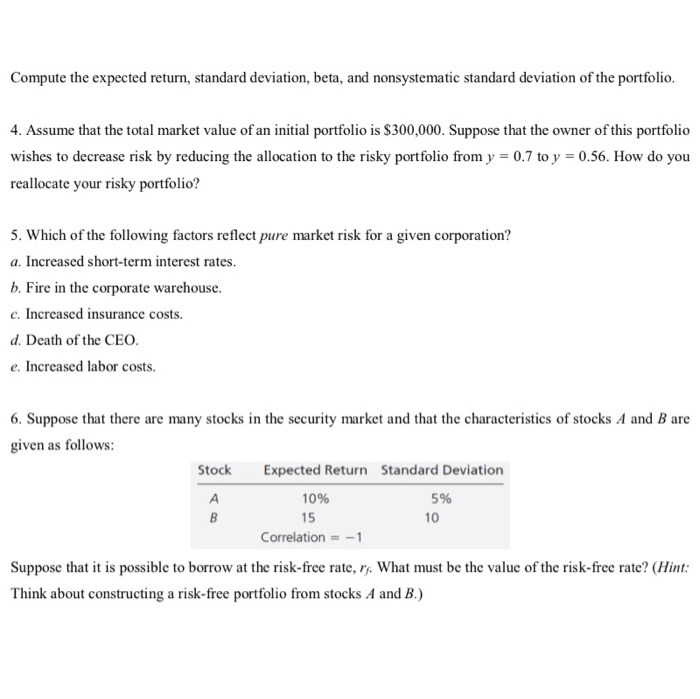Compute the expected return, standard deviation, beta, and nonsystematic standard deviation of the portfolio. 4. Assume that the total market value of an initial portfolio is \$300,000. Suppose that the owner of this portfolio wishes to decrease risk by reducing the allocation to the risky portfolio from y = 0.7 to y = 0.56. How do you reallocate your risky portfolio? 5. Which of the following factors reflect pure market risk for a given corporation? a. Increased short-term interest rates....

• ### (2*5) Consider a market with many risky assets and a risk-free security. Asset’s returns are not...

(2*5) Consider a market with many risky assets and a risk-free security. Asset’s returns are not perfectly correlated. All the CAPM assumptions hold and the market is in equilibrium. The risk-free rate is 5%, the expected return on the market is 15%. Mr. T and Mrs. R are two investors with mean-variance utility functions and different risk-aversion coefficients. They both invest into efficient portfolios composed of the market portfolio and the risk-free security. Mr. T’s portfolio has an expected return...

• ### Consider the following information Expected Standard Portfolio Return Deviation Risk-free 10% 1.0 Market 18 A 16...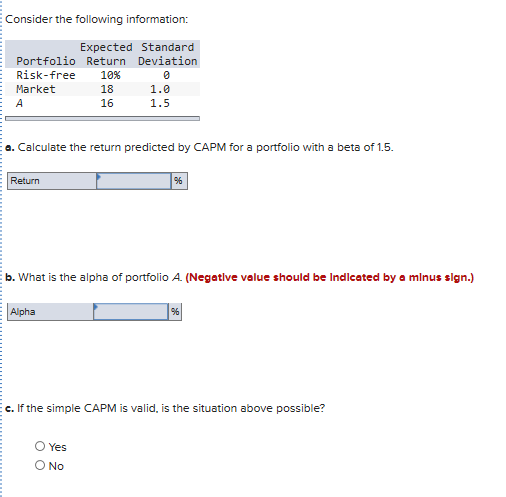Consider the following information Expected Standard Portfolio Return Deviation Risk-free 10% 1.0 Market 18 A 16 1.5 a. Calculate the return predicted by CAPM for a portfolio with a beta of 1.5 Return b. What is the alpha of portfolio A. (Negatlve value should be Indicated by a minus sign.) Alpha c. If the simple CAPM is valid, is the situation above possible? O Yes O No

• ### ve 3.24 The risk-free ering the following investments. free rate is currently 3%, and the market...ve 3.24 The risk-free ering the following investments. free rate is currently 3%, and the market return is 10%. Assume you are consid- Beta Investment 1.5 1.0 0.75 0.0 b. Use the capital asset pricing a. Which investment is most risky? Least risky? b. Use the capital asset pricing model to find the required return on each of the investments. c. Using your findings in part b, draw the security market line. d. On the basis of your findings in...

• ### Parker has a portfolio with a beta of 1.0 and an a porttolio with a beta...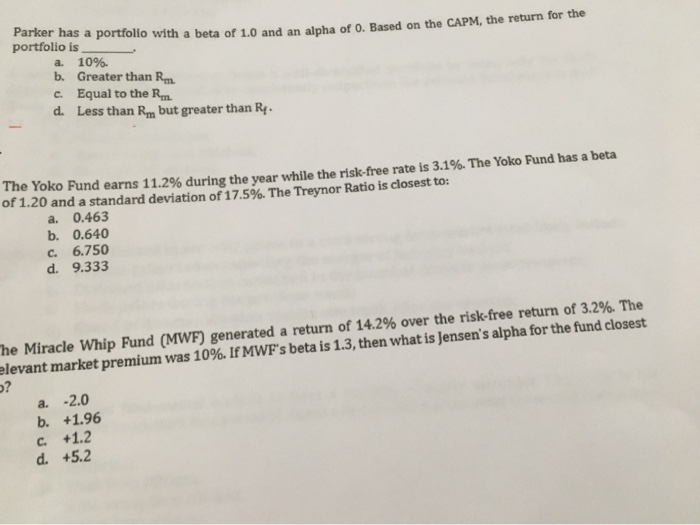Parker has a portfolio with a beta of 1.0 and an a porttolio with a beta of 1.0 and an alpha of 0. Based on the CAPM, the return for the portfolio is a. 10% b. Greater than Rm. C. Equal to the Rm. d. Less than Rm but greater than R. ne TOKO Fund earns 11.2% during the year while the risk-free rate is 3.1%. The Yoko Fund has a beta of 1.20 and a standard deviation of 17.5%....

• ### 1. The standard deviation of market portfolio returns is 15%. The beta of a mutual fund is 1.5. Can the standard deviati...

1. The standard deviation of market portfolio returns is 15%. The beta of a mutual fund is 1.5. Can the standard deviation of mutual fund's returns be 20%? a. Yes b. No 2.  The risk-free rate is 2%. The β of stock 1 is 0.8 while its σ is 15%. The beta of stock 2 is 1.6 while its σ is 45%. Which of the following statements is true in equilibrium? a. The risk premium of stock 2 would be three...